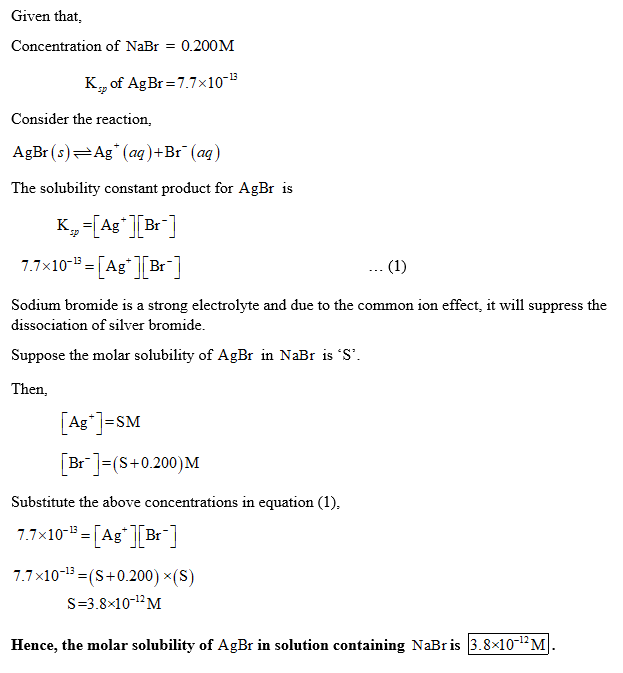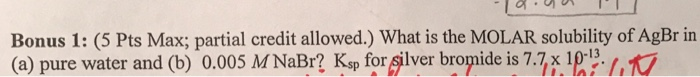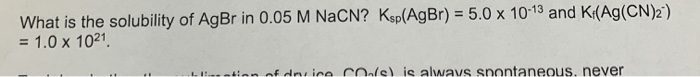# Determine the molar solubility of AgBr in a solution containing 0.200 M NaBr. Ksp (AgBr) = 7.7 × 10-13. a. 3.8 × 10-12...

Determine the molar solubility of AgBr in a solution containing 0.200 M NaBr. Ksp (AgBr) = 7.7 × 10-13.

a. 3.8 × 10-12 M

b. 5.8 × 10-5 M

c. 0.200 M

d. 8.8 × 10-7 M

e.1.54 × 10-13 M##### Add Answer of: Determine the molar solubility of AgBr in a solution containing 0.200 M NaBr. Ksp (AgBr) = 7.7 × 10-13. a. 3.8 × 10-12...
Similar Homework Help Questions
• ### Determine the molar solubility of AgBr in a solution containing 0.150 M NaBr. Ksp (AgBr) = 7.7 × 10-13.

Determine the molar solubility of AgBr in a solution containing 0.150 M NaBr. Ksp (AgBr) = 7.7 × 10-13.

• ### Determine the molar solubility of AgBr in: Ksp (AgBr) = 7.7 x 10^ -13 a. a solution of pure water b. a solution conta...

Determine the molar solubility of AgBr in: Ksp (AgBr) = 7.7 x 10^ -13 a. a solution of pure water b. a solution containing 0.150 M NaBr c. A solution containing 0.25 M NaCL

• ### calculate the molar solubility of AgBr (Ksp = 7.7×10^-13) in a 5.0 x10^-6 M AgNO3 (aq)...

calculate the molar solubility of AgBr (Ksp = 7.7×10^-13) in a 5.0 x10^-6 M AgNO3 (aq) solution.

• ### Determine the molar solubility of BaF2 in a solution containing 0.350 M NaF. Ksp (BaF2) =...

Determine the molar solubility of BaF2 in a solution containing 0.350 M NaF. Ksp (BaF2) = 9.8 × 10-6. a) 4.5 × 10-5 M b) 8.0× 10-5 M c) 3.6 × 10-4 M d) 2.3 × 10-5 M e) 8.2 × 10-9 M

• ### what is the molar solubility of AgBr in 0.10M FeBr3? Ksp(AgBr)= 5.0*10^-13 a. 3.9*10^-14M b. 1.7*10^-12M...

what is the molar solubility of AgBr in 0.10M FeBr3? Ksp(AgBr)= 5.0*10^-13 a. 3.9*10^-14M b. 1.7*10^-12M c. 1.9*10^-11M d. 2.7*10^-10M e. 6.2*10^-12M

• ### Determine the molar solubility of AgBr (Ksp = 5.40e-13) in 2.983 M NH3 if the complex...

Determine the molar solubility of AgBr (Ksp = 5.40e-13) in 2.983 M NH3 if the complex ion [Ag(NH3)2]+ forms with a Kf = 1.70e7. 3.012e-3 0.01136 8.984e-3 7.104e-3

• ### Bonus 1: (5 Pts Max; partial credit allowed.) What is the MOLAR solubility of AgBr in...Bonus 1: (5 Pts Max; partial credit allowed.) What is the MOLAR solubility of AgBr in (a) pure water and (b) 0.005 M NaBr? Ksp for silver bromide is 7.7, x 10 , T

• ### Determine the molar solubility of BaF2 in a solution containing 0.0750 M LiF. Ksp, BaF2 = 1.7 X 10-6. Which one of th...

Determine the molar solubility of BaF2 in a solution containing 0.0750 M LiF. Ksp, BaF2 = 1.7 X 10-6. Which one of these is the correct answer? BaF2 molar solubility = 2.3 x 10-5 M BaF2 molar solubility = 0.0750 M BaF2 molar solubility = 8.5 x 10-7 M BaF2 molar solubility = 1.2 x 10-2 M BaF2 molar solubility = 3.0 x 10-4 M

• ### Determine the molar solubility of BaF2 in a solution containing 0.0750 M LiF. Ksp (BaF2) = 1.7

Determine the molar solubility of BaF2 in a solution containing 0.0750 M LiF. Ksp (BaF2) = 1.7

• ### What is the solubility of AgBr in 0.05 M NaCN? Ksp(AgBr) = 5.0 x 10-13 and...What is the solubility of AgBr in 0.05 M NaCN? Ksp(AgBr) = 5.0 x 10-13 and Kr(Ag(CN)2') = 1.0 x 1021 LIS-ntion of drico Coel is always spontaneous, never

Free Homework App